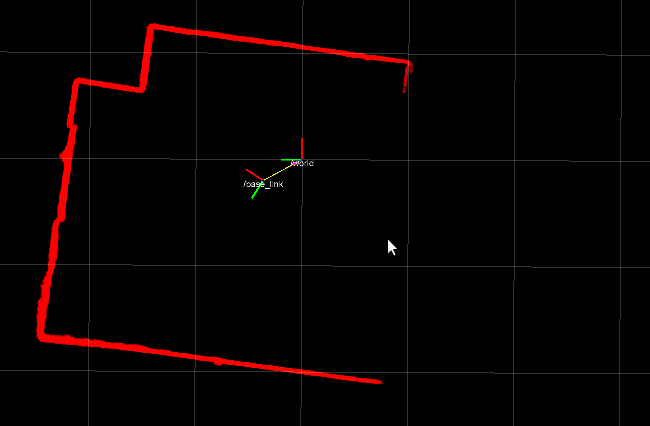# Don't compute it twice

The example I am going to present will make some of you react like this:I say this because it will be absolutely obvious... in retrospective.

On the other hand, I have seen this same code being used in multiple open source projects.

Projects with hundreds of Github stars missed this (apparently obvious) opportunity for optimization.

A notable example is: Speed up improvement for laserOdometry and scanRegister (20%)

## 2D transforms

Let's consider this code:

``````double x1 = x*cos(ang) - y*sin(ang) + tx;
double y1 = x*sin(ang) + y*cos(ang) + ty;
``````

People with a trained eye (and a little of trigonometric background) will immediately recognize the [affine transform of a 2D point], commonly used in computer graphics and robotics.

Don't you see anything we can do better? Of course:

``````const double Cos = cos(angle);
const double Sin = sin(angle);
double x1 = x*Cos - y*Sin + tx;
double y1 = x*Sin + y*Cos + ty;
``````

The cost of trigonometric functions is relatively high and there is absolutely no reason to compute twice the same value. The latter code will be 2x times faster then the former, because the cost of multiplication and sum is really low compared with `sin()` and `cos()`.

In general, if the number of potential angles you need to test is not extremely high, consider to use look-up-table where you can store pre-computed values.

This is the case, for instance, of laser scan data, that needs to be converted from polar coordinates to cartesian ones.A naive implementation would invoke trigonometric functions for each point (in the order of thousands per seconds).

``````// Conceptual operation (inefficient)
// Data is usually stored in a vector of distances
std::vector<double> scan_distance; // the input
std::vector<Pos2D> cartesian_points; // the output

cartesian_points.reserve( scan_distance.size() );

for(int i=0; i<scan_distance.size(); i++)
{
const double dist = scan_distance[i];
const double angle = angle_minimum + (angle_increment*i);
double x = dist*cos(angle);
double y = dist*sin(angle);
cartesian_points.push_back( Pos2D(x,y) );
}
``````

But we should consider that:

• angle_minimum and angle_increment are constants that never change.
• the size of the scan_distance is constant too (not its content, of course).

This is the perfect example where a LUT makes sense and will dramatically improve performance.

``````//------ To do only ONCE -------
std::vector<double> LUT_cos;
std::vector<double> LUT_sin;

for(int i=0; i<scan_distance.size(); i++)
{
const double angle = angle_minimum + (angle_increment*i);
LUT_cos.push_back( cos(angle) );
LUT_sin.push_back( sin(angle) );
}

// ----- The efficient scan conversion ------
std::vector<double> scan_distance;
std::vector<Pos2D> cartesian_points;

cartesian_points.reserve( scan_distance.size() );

for(int i=0; i<scan_distance.size(); i++)
{
const double dist = scan_distance[i];
double x = dist*LUT_cos[i];
double y = dist*LUT_sin[i];
cartesian_points.push_back( Pos2D(x,y) );
}
``````

# Lessons to take home

This is a simple example; what you should learn from this is that, whenever an operation is expensive to compute (SQL queries, stateless mathematical operations), you should consider to use a cached value and or to build a look-up-table.

But, as always, measure first to be sure that the optimization is actually relevant ;)